## python使用matplotlib绘图 — barChart

matploitlib画图的各种各样的源码以及图样:

http://matplotlib.org/gallery.html

matplotlib 是python最著名的绘图库，它提供了一整套和matlab相似的命令API，十分适合交互式地进行制图。而且也可以方便地将它作为绘图控件，嵌入GUI应用程序中。它的文档相当完备，并且 Gallery页面 中有上百幅缩略图，打开之后都有源程序。因此如果你需要绘制某种类型的图，只需要在这个页面中浏览/复制/粘贴一下，基本上都能搞定。  —–引用自：http://hyry.dip.jp/pydoc/matplotlib_intro.html

import matplotlib.pyplot as plt

plt.bar(left = 0,height = 1)
plt.show()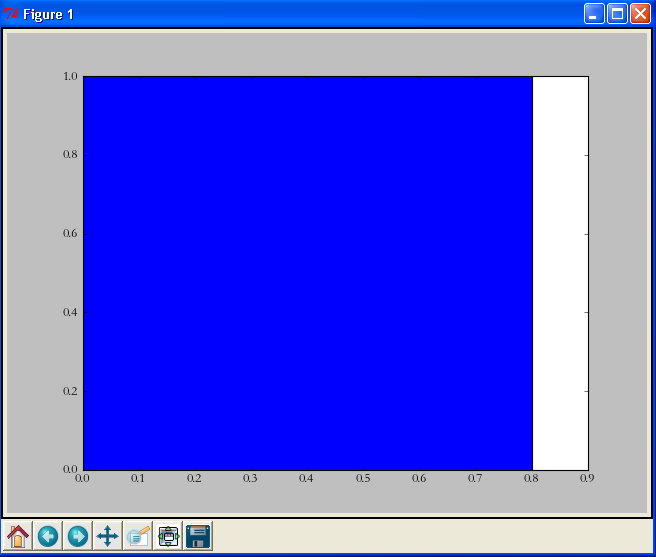• left：柱形的左边缘的位置，如果我们指定1那么当前柱形的左边缘的x值就是1.0了
• height：这是柱形的高度，也就是Y轴的值了
left，height除了可以使用单独的值（此时是一个柱形），也可以使用元组来替换（此时代表多个矩形）。例如，下面的例子：
import matplotlib.pyplot as plt

plt.bar(left = (0,1),height = (1,0.5))
plt.show()

`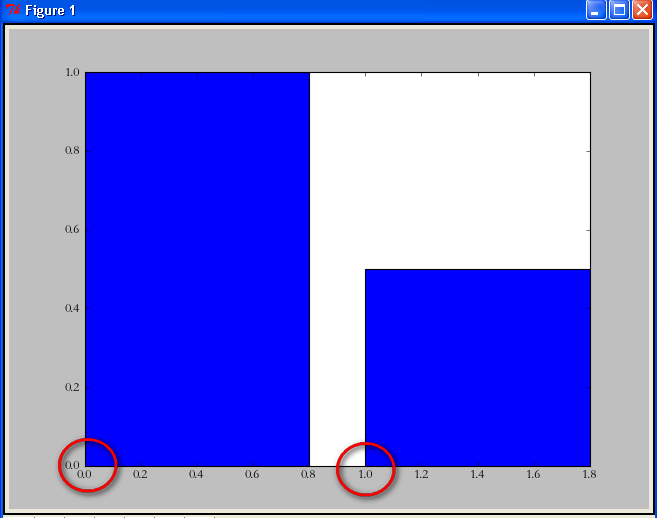`

import matplotlib.pyplot as plt

plt.bar(left = (0,1),height = (1,0.5),width = 0.35)
plt.show()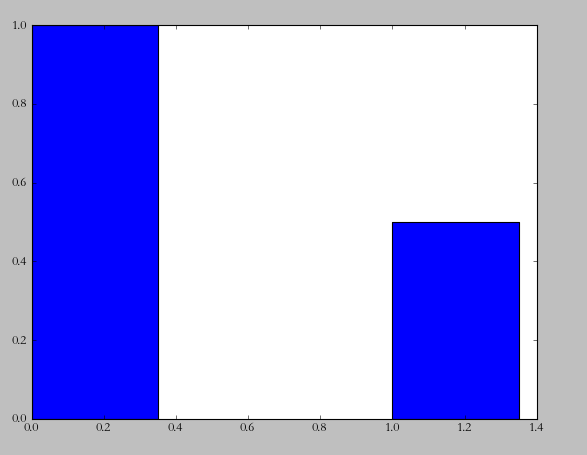import matplotlib.pyplot as plt

plt.xlabel(u’性别’)
plt.ylabel(u’人数’)
plt.bar(left = (0,1),height = (1,0.5),width = 0.35)
plt.show()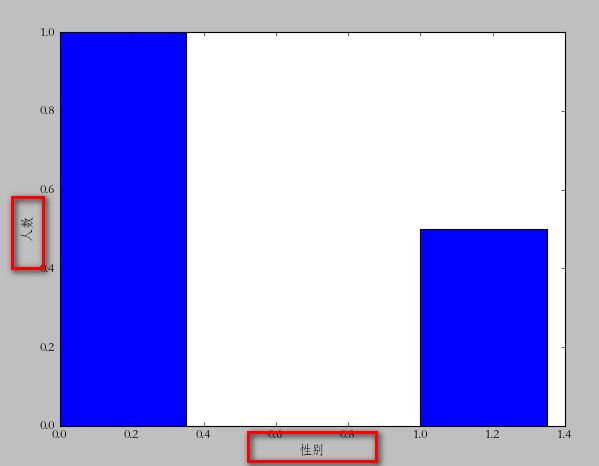import matplotlib.pyplot as plt

plt.xlabel(u’性别’)
plt.ylabel(u’人数’)

plt.xticks((0,1),(u’男’,u’女’))

plt.bar(left = (0,1),height = (1,0.5),width = 0.35)

plt.show()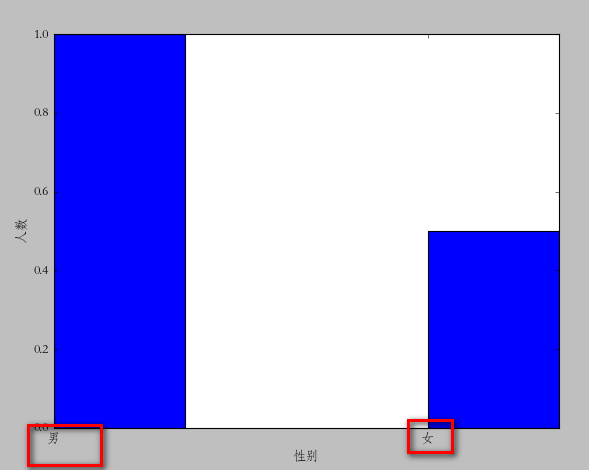plt.xticks的用法和我们前面说到的left,height的用法差不多。如果你有几个bar，那么就是几维的元组。第一个是文字的位置，第二个是具体的文字说明。不过这里有个问题，很显然我们指定的位置有些“偏移”，最理想的状态应该在每个矩形的中间。你可以更改(0,1)=>( (0+0.35)/2 ,(1+0.35)/2 )不过这样比较麻烦。我们可以通过直接指定bar方法里面的align=”center”就可以让文字居中了。
import matplotlib.pyplot as plt

plt.xlabel(u’性别’)
plt.ylabel(u’人数’)

plt.xticks((0,1),(u’男’,u’女’))

plt.bar(left = (0,1),height = (1,0.5),width = 0.35,align=”center”)

plt.show()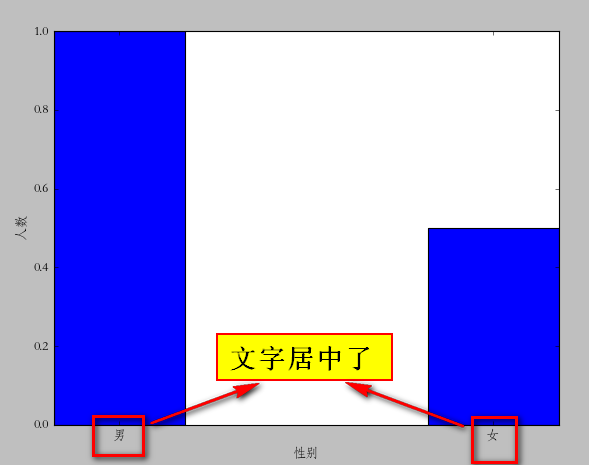plt.title(u”性别比例分析”)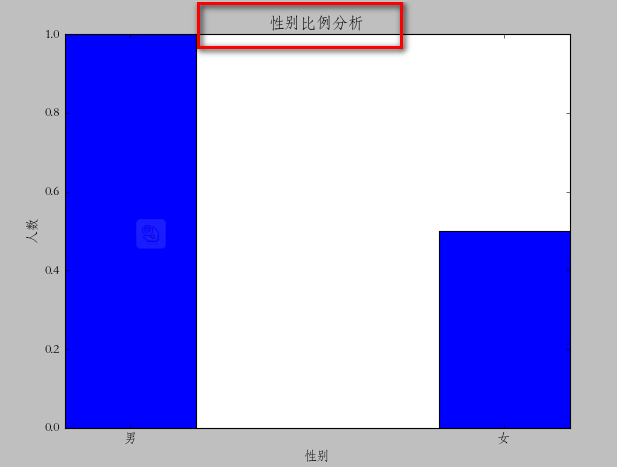import matplotlib.pyplot as plt

plt.xlabel(u’性别’)
plt.ylabel(u’人数’)

plt.title(u”性别比例分析”)
plt.xticks((0,1),(u’男’,u’女’))
rect = plt.bar(left = (0,1),height = (1,0.5),width = 0.35,align=”center”)

plt.legend((rect,),(u”图例”,))

plt.show()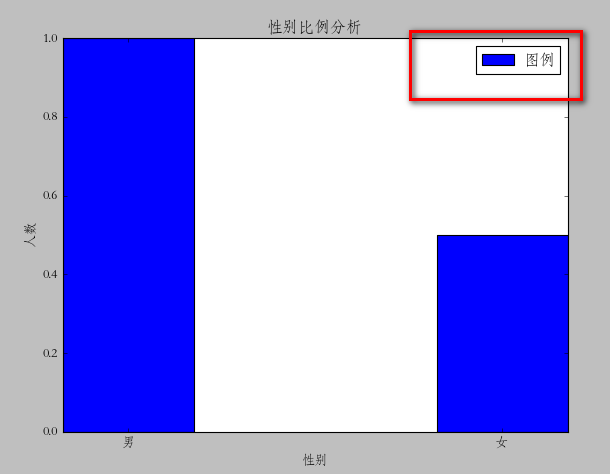def autolabel(rects):
for rect in rects:
height = rect.get_height()
plt.text(rect.get_x()+rect.get_width()/2., 1.03*height, ‘%s’ % float(height))

import matplotlib.pyplot as plt

def autolabel(rects):
for rect in rects:
height = rect.get_height()
plt.text(rect.get_x()+rect.get_width()/2., 1.03*height, ‘%s’ % float(height))

plt.xlabel(u’性别’)
plt.ylabel(u’人数’)

plt.title(u”性别比例分析”)
plt.xticks((0,1),(u’男’,u’女’))
rect = plt.bar(left = (0,1),height = (1,0.5),width = 0.35,align=”center”)

plt.legend((rect,),(u”图例”,))
autolabel(rect)

plt.show()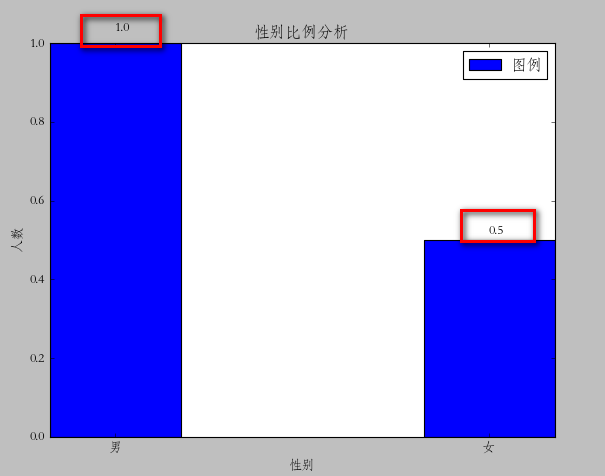rect = plt.bar(left = (0,1),height = (1,0.5),width = 0.35,align=”center”,yerr=0.000001)

`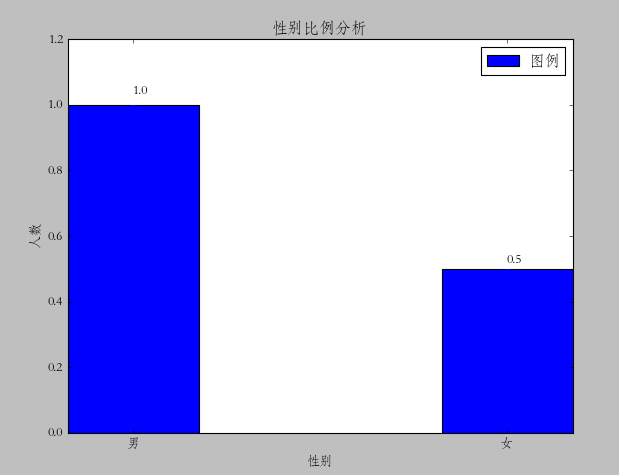`

## MATLAB的符号计算

（一） 定义符号变量

1、sym函数
sym函数的主要功能是创建符号变量，以便进行符号运算，也可以用于创建符号表达式或符号矩阵。用sym函数创建符号变量的一般格式为：

x = sym(‘x’)

 1 2 3 4 5 6 7 8 9 `a=sym(‘a’); %定义‘a’为符号运算量，输出变量名为a` `b=sym(‘b’);` `x=sym(‘x’);` `y=sym(‘y”);   ` `[x,y]=solve(‘a*x-b*y=1’, ‘a*x+b*y=5’, ‘x’, ‘y’) ` `                                ``%以a,b为符号常数，x,y为符号变量` `即可得到方程组的解：` `x =3/a` `y =2/b`

（一） 定义符号变量

【例2】已知一复数表达式 z=x+i*y, 试求其共轭复数,并求该表达式与其共轭复数乘积的多项式。

x=sym(‘x’,’real’);
y=sym(‘y’,’real’);

z=x+i*y; %定义复数表达式
conj(z); %求共轭复数
expand(z*conj(z)) %求表达式与其共轭复数乘积的

ans =
x^2+y^2

x = sym(‘x’,’unreal’)

2、syms函数
syms函数的功能与sym函数类似。syms函数可以在一个语句中同时定义多个符号变量，其一般格式为：
syms arg1 arg2 …argN

（二）默认符号变量

f=ax2+bx+c

f=xn
g=sin(at+b)（四） 生成符号函数

syms a b c x y              %定义符号运算量
fxy=(a*x^2+b*y^2)/c^2      %生成符号函数

 1 2 3 4 5 6 7 8 9 `【例4】定义一个符号函数 fxy=(a*x2+b*y2)/c2 ，分别求该函数对x、y的导数和对x的积分。` `syms a b c x y            %定义符号变量` `fxy=(a*x^2+b*y^2)/c^2;  %生成符号函数  ` `diff(fxy,x)             %符号函数fxy对x求导数` `ans =2*a*x/c^2` `diff(fxy, y)            %符号函数fxy对y求导数    ` `ans =2*b*y/c^2` `int``(fxy, x)             %符号函数fxy对x求积分` `ans =1/c^2*(1/3*a*x^3+b*y^2*x)`

（五）标准代数运算

f= ‘ 2*x^2+3*x-5 ‘   %  define the symbolic expression
>>f=
2*x^2+3*x-5
>> g= ‘ x^2-x+7 ‘
g=
x^2-x+7

>> symadd(f，g)  %  find an expression for f+g
ans= 3*x^2+2*x+2
>> symsub(f，g)  %  find an expression for f-g
ans= x^2+4*x-12
>> symmul(f，g)  %  find an expression for f*g
ans= (2*x^2+3*x-5)*(x^2-x+7)
>> symdiv(f，g)  %  find an expression for f/g
ans= (2*x^2+3*x-5)/(x^2-x+7)
>> sympow(f， ‘ 3*x ‘ )  %  the same as sym(A)^sym(B)
ans= (2*x^2+3*x-5)^3*x

## matlab符号函数及其运算0基础入门

matlab有强大的符号运算的功能。在符号运算下可以对方程，方程组进行求导，求偏导，求积分等复杂运算，加减乘除等简单运算更加易如反掌。

1.符号函数的定义：

syms x y   %定义两个符号,x和y

2.使用x,y定义两个方程如下：

>> f1 = 2*x^2 + x^y + x*y + 1;
>> f2 = exp(x^y) + x*y + 100;

%这是两个何其复杂的方程啊

>> F = [f1;f2];
>> F

F =

x*y + x^y + 2*x^2 + 1
exp(x^y) + x*y + 100

3. 求偏微分
>> dFdx = diff(F,x)

dFdx =

4*x + y + x^(y – 1)*y
y + x^(y – 1)*y*exp(x^y)

>>

4. 符号赋值求具体值：
>> B = [diff(f1,x),diff(f1,y);diff(f2,x),diff(f2,y)]

B =

[    4*x + y + x^(y – 1)*y,          x + x^y*log(x)]
[ y + x^(y – 1)*y*exp(x^y), x + x^y*exp(x^y)*log(x)]

>> x0 = 1;y0 = 4;
>> B0 = subs(B,{x,y},{x0,y0})

B0 =

12.0000    1.0000
14.8731    1.0000

>>

5. 再说一个积分的例子吧

>>
>>
>> f3 = 3*x + 4*y + 100;
>> f3_x = int(f3,x)  %对f3函数对x求积分

f3_x =

(3*x + 4*y + 100)^2/6

>> %将f3_x对x求导数确实等于f3，即验证了int函数的求积分功能

num := 50;
for i from 1 to num do
x(i) := (i*PI)/num;
end_for;
for i from 1 to num do
y(i) := sin(x(i));
z(i) := cos(x(i));
end_for;

// f1 := plot::Line2d([x(i),y(i)],[x(i+1),y(i+1)],Color=RGB::Blue) \$ i=1..(num-1) ;
// f2 := plot::Line2d([x(j),z(j)],[x(j+1),z(j+1)],Color=RGB::Red) \$ j=1..(num-1) ;
// plot(f1,f2);
f3 := plot::Polygon2d([[x(i),y(i)] \$i = 1..(num-1)],Closed = FALSE);
// S1 = plot::Scene2d(f3,Filled = TRUE,FillStyle = Winding);
plot(f3,Filled = TRUE,FillStyle = Winding);

Closed = TRUE 或者 Closed = FALSE来设置是否要把这个多边形弄成封闭的多边形。f4 := plot::Polygon2d([[x(1),0],[x(i),z(i)] \$i=1..(num),[x(num),0]],Closed = FALSE,Color=RGB::White);
f5 := plot::Polygon2d([[x(i),z(i)] \$i=1..(num)],Closed = FALSE,Color=RGB::Red);

f4, f5效果图：f3,f4,f5的赋值再次写在这里：

f3 := plot::Polygon2d([[x(i),y(i)] \$i = 1..(num-1)],Closed = FALSE,Color=RGB::Blue,FillPattern = FDiagonalLines); //设置成反斜线
f4 := plot::Polygon2d([[x(1),0],[x(i),z(i)] \$i=1..(num),[x(num),0]],Closed = FALSE,Color=RGB::White);
f5 := plot::Polygon2d([[x(i),z(i)] \$i=1..(num)],Closed = FALSE,Color=RGB::Red);
plot(f3,f4,f5,Filled = TRUE,FillStyle = Winding ,Layout = Horizontal);f3 := plot::Polygon2d([[x(i),y(i)] \$i = 1..(num-1)],Closed = FALSE,Color=RGB::Blue,FillPattern = FDiagonalLines); //设置成反斜线
//f4 := plot::Polygon2d([[x(1),0],[x(i),z(i)] \$i=1..(num),[x(num),0]],Closed = FALSE,Color=RGB::White);
f5 := plot::Polygon2d([[x(i),z(i)] \$i=1..(num)],Closed = FALSE,Color=RGB::Red);
//plot(f3,f4,f5);
f6 := plot::Polygon2d([[x(i),y(i)] \$i=20..(30),[x(abs(j-30)),z(abs(j-30))] \$j = 0..10],Closed = TRUE,Color = RGB::Green,Filled = TRUE);
plot(f3,f5,f6);``` num := 50; for i from 1 to num do x(i) := (i*PI)/num; end_for; for i from 1 to num do y(i) := sin(x(i)); z(i) := cos(x(i)); end_for; ```

plot(plot::Line2d([x(i),y(i)],[x(i+1),y(i+1)]) \$ i=1..(num-1),plot::Line2d([x(j),z(j)],[x(j+1),z(j+1)]) \$ j=1..(num-1),Color=RGB::Blue);f1 := plot::Line2d([x(i),y(i)],[x(i+1),y(i+1)],Color=RGB::Blue) \$ i=1..(num-1) ;
f2 := plot::Line2d([x(j),z(j)],[x(j+1),z(j+1)],Color=RGB::Red) \$ j=1..(num-1) ;

plot(f1,f2)plot::Listplot 来对数组进行画线。

y2 := array(1..num);
for i from 1 to num do
y2[i] := sin(x(i));
end_for;
plot( plot::Listplot(y2,PointsVisible=FALSE) )### 一个例子p1:=piecewise([x=0.99,1]):
f1:=plot::Function2d(p1,x=0.95 .. 1.01,LineColor=RGB::Black):
f2:=plot::Function2d(1.01/x,x=0.95 .. 1.01,LineColor=RGB::Black):
f3:=plot::Function2d(0.98/x,x=0.95 .. 0.98,LineColor=RGB::Black,LineStyle=Dashed):
f4:=plot::Function2d(1.02/x,x=0.95 .. 1.02,LineColor=RGB::Black,LineStyle=Dashed):
g:=plot(f1,f2,f3,f4,plot::Hatch(f1,f2,x=0.95 .. 1.01,FillColor=RGB::Black),
plot::Rectangle(0.95..1.05,1..1.1,LineColor=RGB::Black)):

• 在输入命令时，如果需要换行，按Ctrl+Enter；直接按Enter的话，就输出结果。
• 如果一次要运行多个命令，需用”:”把命令分隔开。

## matlab中patch函数的用法

patch

patch(X,Y,C)

patch(X,Y,Z,C)

patch(FV)

patch(…’PropertyName’,propertyvalue…)

patch(‘PropertyName’,propertyvalue,…)

handle = patch(…)

patch是个底层的图形函数，用来创建补片图形对象。一个补片对象是由其顶点坐标确定的一个或多个多边形。用户可以指定补片对象的颜色和灯光。

patch（X,Y,C）

添加已填充的二维补片到当前坐标轴。X和Y中的元素指定了多边形的定点。如果X和Y是矩阵，MATLAB将每一列生成一个多边形。C决定了补片的颜色，它可以是单个的ColorSpec，每个表面一个颜色，或每个定点一个颜色。如果C是1*3的向量，它将被看成是RGB三元组，直接指定颜色。

patch（X,Y,Z,C）

创建三维坐标下的补片。

patch（FV）

使用结构体FV来创建补片。FV包含如下的结构域：vertices、faces以及facevertexdata（可选）。这些结构域对应补片对象的Vertices、Faces和FaceVerticxCData属性。

patch（‘PropertyName’，propertyvalue，…）

利用指定的属性/值参数对来指定补片对象的所有属性。除非用户显式的指定FaceColor和EdgeColor的值，否则，MATLAB会使用缺省的属性值。该调用格式允许用户使用Faces和Vertices属性值来定义补片。

handle=patch（…）

返回创建的补片对象的句柄。

不像fill或area这样的高层创建函数，patch并不检查图形窗口的设置以及坐标轴的NextPlot属性，它仅仅将补片对象添加到当前坐标轴。

如果坐标数据不能定义封闭的多边形，patch函数自动使多边形封闭。数据能定理凹面或交叉的多边形。然而，如果单个补片面的边缘相互交叉，得到的面可能不会完全填充。在这种情况下，最好将面分解为更小的多边形。

你可以注明这些属性为：属性名字和属性值成对形式、结构矩阵和细胞矩阵。

其中有两个属性是用来说明颜色的：

CData：用在说明X、Y、Z坐标时（XData、YData、ZData）

FaceVertexCData：用在说明顶点或联接矩阵（Vertices和Faces）

CData和FaceVertexCData属性接受这样的颜色数据：索引值、真彩色（RGB）值。（CData、FaceVertexCData）

索引颜色数据可以表示整个色图的直接索引值或线性映射到整个色图的刻度值（caxis）。CDataMapping 决定了matlab如何翻译索引颜色数据。patch的颜色说明如下：

所有面用同一个颜色；

一个面一个颜色，即平面着色；

一个顶点一个颜色，即插值着色；

下面的表格说明了matlab如何解释由CData、FaceVertexCData定义的颜色数据。

[X,Y,Z]                    CData

索引值            真彩色

m*n             标量              1*1*3        所有面用同一个颜色，边缘用同一个颜色

m*n            1*n（n>=4）        1*n*3        一个面一个颜色，边缘用一个颜色
m*n              m*n             m*（n-3）     一个顶点一个颜色，面可以是平滑的一个颜色的或插值的，边缘也可以是平滑的或插值的。

顶点         面             FaceVertexCData

索引值          真彩色

m*n          k*3         标量            1*3

m*n          k*3          k*1            k*3               同上

m*n          k*3          m*1            m*3

该例中使用两种不同的方法来创建一个补片对象：

指定x、y和z坐标以及颜色数据（XData、YData和ZData以及CData属性）

指定顶点，连接矩阵以及颜色矩阵（Vertices、Faces、FaceVertexCData以及FaceColor属性）

1、指定x、y和z坐标

x=[0 0;0 1;1 1];

y=[1 1;2 2;2 1];

z=[1 1;1 1;1 1];

tcolor(1,1,1:3)=[1 1 1];

tcolor(1,2,1:3)=[.7 .7 .7];

patch(x,y,z,tcolor)

2、指定定点和表面

Vertices属性包含定义补片的每一个定点的坐标，Faces属性确定如何连接这些顶点来组成不判的每一个表面。在本例中，两个顶点位于同一个位置，因此需要指定六个顶点。每一样包含定点的x、y和z坐标。
vert=[0 1 1;0 2 1;1 2 1;1 1 1];

有两个表面，按照指定的顺序连接定点而成：

fac=[1 2 3；1 3 4]；

指定表面的颜色，指定包含两个RGB颜色的2*3的矩阵；

tcolor=[1 1 1；.7 .7 .7];

用户必须设置FaceColor属性为flat，通过指定Faces、Vertices、FaceVertexCData以及FaceColor属性来创建

补片对象：

patch（’Faces’,fac,’Vertices’,vert,’FaceVertexCData’,tcolor,’FaceColor’,’flat’）

结果如上图所示。。。。。。。

## 图像处理函数详解——imdilate

IM2 = imdilate(IM,NHOOD)
IM2 = imdilate(IM,SE,PACKOPT)
IM2 = imdilate(IM,SE) 膨胀灰度，二值，压缩二值图像IM，返回IM2。参数SE为由strel函数返回的结构元素或者结构元素对象组。
IM2 = imdilate(IM,NHOOD)膨胀图像IM，这里NHOOD是定义结构元素邻域0和1的矩阵。
IM2 = imdilate(IM,SE,PACKOPT)定义IM是否是一个压缩的二值图像。

se = strel(‘ball’,5,5);
I2 = imdilate(I,se);
imshow(I), title(‘Original’)

figure, imshow(I2), title(‘Dilated’)

# 海量数据处理专题（一）——开篇

下面的方法是我对海量数据的处理方法进行了一个一般性的总结，当然这些方法可能并不能完全覆盖所有的问题，但是这样的一些方法也基本可以处理绝大多数遇到的问题。下面的一些问题基本直接来源于公司的面试笔试题目，方法不一定最优，如果你有更好的处理方法，欢迎与我讨论。

本贴从解决这类问题的方法入手，开辟一系列专题来解决海量数据问题。拟包含 以下几个方面。

1. Bloom Filter
2. Hash
3. Bit-Map
4. 堆(Heap)
5. 双层桶划分
6. 数据库索引
7. 倒排索引（Inverted Index）
8. 外排序
9. Trie树
10. MapReduce

在这些解决方案之上，再借助一定的例子来剖析海量数据处理问题的解决方案。

# 海量数据处理专题（二）——Bloom Filter

【什么是Bloom Filter】
Bloom Filter是一种空间效率很高的随机数据结构，它利用位数组很简洁地表示一个集合，并能判断一个元素是否属于这个集合。Bloom Filter的这种高效是有一定代价的：在判断一个元素是否属于某个集合时，有可能会把不属于这个集合的元素误认为属于这个集合（false positive）。因此，Bloom Filter不适合那些“零错误”的应用场合。而在能容忍低错误率的应用场合下，Bloom Filter通过极少的错误换取了存储空间的极大节省。 这里有一篇关于Bloom Filter的详细介绍，不太懂的博友可以看看。
【适用范围】

【基本原理及要点】

【扩展】
Bloom filter将集合中的元素映射到位数组中，用k（k为哈希函数个数）个映射位是否全1表示元素在不在这个集合中。Counting bloom filter（CBF）将位数组中的每一位扩展为一个counter，从而支持了元素的删除操作。Spectral Bloom Filter（SBF）将其与集合元素的出现次数关联。SBF采用counter中的最小值来近似表示元素的出现频率。

【问题实例】

# 海量数据处理专题（三）——Hash

【什么是Hash】
Hash，一般翻译做“散列”，也有直接音译为“哈希”的，就是把任意长度的输入（又叫做预映射， pre-image），通过散列算法，变换成固定长度的输出，该输出就是散列值。这种转换是一种压缩映射，也就是，散列值的空间通常远小于输入的空间，不同的输入可能会散列成相同的输出，而不可能从散列值来唯一的确定输入值。简单的说就是一种将任意长度的消息压缩到某一固定长度的消息摘要的函数。
HASH主要用于信息安全领域中加密算法，它把一些不同长度的信息转化成杂乱的128位的编码,这些编码值叫做HASH值. 也可以说，hash就是找到一种数据内容和数据存放地址之间的映射关系。1，除法散列法

index = value % 16

2，平方散列法

index = (value * value) >> 28

3，斐波那契（Fibonacci）散列法

1，对于16位整数而言，这个乘数是40503
2，对于32位整数而言，这个乘数是2654435769
3，对于64位整数而言，这个乘数是11400714819323198485

i ndex = (value * 2654435769) >> 28【适用范围】

【基本原理及要点】
hash函数选择，针对字符串，整数，排列，具体相应的hash方法。

【扩展】
d-left hashing中的d是多个的意思，我们先简化这个问题，看一看2-left hashing。2-left hashing指的是将一个哈希表分成长度相等的两半，分别叫做T1和T2，给T1和T2分别配备一个哈希函数，h1和h2。在存储一个新的key时，同 时用两个哈希函数进行计算，得出两个地址h1[key]和h2[key]。这时需要检查T1中的h1[key]位置和T2中的h2[key]位置，哪一个 位置已经存储的（有碰撞的）key比较多，然后将新key存储在负载少的位置。如果两边一样多，比如两个位置都为空或者都存储了一个key，就把新key 存储在左边的T1子表中，2-left也由此而来。在查找一个key时，必须进行两次hash，同时查找两个位置。
【问题实例】
1).海量日志数据，提取出某日访问百度次数最多的那个IP。
IP的数目还是有限的，最多2^32个，所以可以考虑使用hash将ip直接存入内存，然后进行统计。

# 海量数据处理专题（四）——Bit-map

【什么是Bit-map】C代码

``` 1     //定义每个Byte中有8个Bit位
2     #include ＜memory.h＞
3     #define BYTESIZE 8
4     void SetBit(char *p, int posi)
5     {
6         for(int i=0; i ＜ (posi/BYTESIZE); i++)
7         {
8             p++;
9         }
10
11         *p = *p|(0x01＜＜(posi%BYTESIZE));//将该Bit位赋值1
12         return;
13     }
14
15     void BitMapSortDemo()
16     {
17         //为了简单起见，我们不考虑负数
18         int num[] = {3,5,2,10,6,12,8,14,9};
19
20         //BufferLen这个值是根据待排序的数据中最大值确定的
21 //待排序中的最大值是14，因此只需要2个Bytes(16个Bit)
22 //就可以了。
23         const int BufferLen = 2;
24         char *pBuffer = new char[BufferLen];
25
26         //要将所有的Bit位置为0，否则结果不可预知。
27         memset(pBuffer,0,BufferLen);
28         for(int i=0;i＜9;i++)
29         {
30             //首先将相应Bit位上置为1
31             SetBit(pBuffer,num[i]);
32         }
33
34         //输出排序结果
35         for(int i=0;i＜BufferLen;i++)//每次处理一个字节(Byte)
36         {
37             for(int j=0;j＜BYTESIZE;j++)//处理该字节中的每个Bit位
38             {
39                 //判断该位上是否是1，进行输出，这里的判断比较笨。
40 //首先得到该第j位的掩码（0x01＜＜j），将内存区中的
41 //位和此掩码作与操作。最后判断掩码是否和处理后的
42 //结果相同
43                 if((*pBuffer&(0x01＜＜j)) == (0x01＜＜j))
44                 {
45                     printf("%d ",i*BYTESIZE + j);
46                 }
47             }
48             pBuffer++;
49         }
50     }
51
52     int _tmain(int argc, _TCHAR* argv[])
53     {
54         BitMapSortDemo();
55         return 0;
56     }```

【适用范围】

【基本原理及要点】

【扩展】

Bloom filter可以看做是对bit-map的扩展

【问题实例】

1)已知某个文件内包含一些电话号码，每个号码为8位数字，统计不同号码的个数。

8位最多99 999 999，大概需要99m个bit，大概10几m字节的内存即可。 （可以理解为从0-99 999 999的数字，每个数字对应一个Bit位，所以只需要99M个Bit==1.2MBytes，这样，就用了小小的1.2M左右的内存表示了所有的8位数的电话）

2)2.5亿个整数中找出不重复的整数的个数，内存空间不足以容纳这2.5亿个整数。

# 海量数据处理专题（五）——堆

【什么是堆】

1）每个节点的值都大于（或者都小于，称为最小堆）其子节点的值
2）树是完全平衡的，并且最后一层的树叶都在最左边【适用范围】

【基本原理及要点】

【扩展】

【问题实例】
1)100w个数中找最大的前100个数。

# 海量数据处理专题（六）

【什么是双层桶】

【适用范围】

【基本原理及要点】

【扩展】

【问题实例】
1).2.5亿个整数中找出不重复的整数的个数，内存空间不足以容纳这2.5亿个整数。

2).5亿个int找它们的中位数。

3).现在有一个0-30000的随机数生成器。请根据这个随机数生成器，设计一个抽奖范围是0-350000彩票中奖号码列表，其中要包含20000个中奖号码。

# 海量数据处理专题（七）——数据库索引及优化

### 数据库索引

#### 概述B树索引-Sql Server索引方式

### 数据库优化第三，切表，切表也是一种比较流行的数据库优化法。分表包括两种方式：横向分表和纵向分表，其中，横向分表比较有使用意义，故名思议，横向切表就是指把记录分到不同的表中，而每条记录仍旧是完整的（纵向切表后每条记录是不完整的），例如原始表中有100条记录，我要切成2个表，那么最简单也是最常用的方法就是ID取摸切表法，本例中，就把ID为1,3,5,7。。。的记录存在一个表中，ID为2,4,6,8,。。。的记录存在另一张表中。虽然横向切表可以减少查询强度，但是它也破坏了原始表的完整性，如果该表的统计操作比较多，那么就不适合横向切表。横向切表有个非常典型的用法，就是用户数据：每个用户的用户数据一般都比较庞大，但是每个用户数据之间的关系不大，因此这里很适合横向切表。最后，要记住一句话就是：分表会造成查询的负担，因此在数据库设计之初，要想好是否真的适合切表的优化：# VSM检索模型VSM模型示例

PS:为了简单起见，这里分词的粒度很大。VSM模型公式

# 倒排索引

• 一条记录的水平反向索引（或者反向档案索引）包含每个引用单词的文档的列表。
• 一个单词的水平反向索引（或者完全反向索引）又包含每个单词在一个文档中的位置。1.左侧的索引表如何建立?怎么做才能最高效？

2.如何快速的添加删除更新索引？

3.那么多的海量文档，如果存储呢？有么有什么备份策略呢？

————————————————————————————————————————————-

## Matlab 把数字产生001、00n这样的字符串 | num2str用法 |sprintf用法 |int2str用法

Matlab 把数字产生001、00n这样的字符串 | num2str用法 |sprintf用法 |int2str用法

Matlab把数字转变成str，可以用num2str, sprintf, int2str等

sprintf:

``` sprintf```

``` Format data into string Syntax str = sprintf(format, A, ...) [str, errmsg] = sprintf(format, A, ...) Description str = sprintf(format, A, ...) applies the format to all elements of array A and any additional array arguments in column order, and returns the results to string str. ```

```[str, errmsg] = sprintf(format, A, ...) returns an error message string when the operation is unsuccessful. Otherwise, errmsg is empty. ```
num2str:
``` num2str```

``` Convert number to string Syntax str = num2str(A) str = num2str(A, precision) str = num2str(A, format) Description The num2str function converts numbers to their string representations. This function is useful for labeling and titling plots with numeric values. str = num2str(A) converts array A into a string representation str. Converted floating-point values have a maximum of four digits of precision, and an exponent if required. For integer values, num2str returns the exact string representation of the value. str = num2str(A, precision) converts the array A into a string representation str with the maximum number of digits specified by precision. str = num2str(A, format) converts array A using the supplied format, as described on the fprintf function reference page. By default, num2str displays floating point values using the '%11.4g' format (four significant digits in exponential or fixed-point notation, whichever is shorter). Remarks ```

```Unlike fprintf, the num2str function trims any leading spaces from a string, even when used with the space character flag. For example, num2str(42.67, '% 10.2f') returns a 1-by-5 character array '42.67'. ```
num2str(1.33333,’%.3d’) 把整数部分格式化成3位精度 1.33e000
num2str(1.333333,’%.3f’) 把小数部分格式化成3位精度 1.333
num2str(1,’%.3d’) 如果是一个整数没有小数部分，对整数部分格式化为3位精度就变为：001